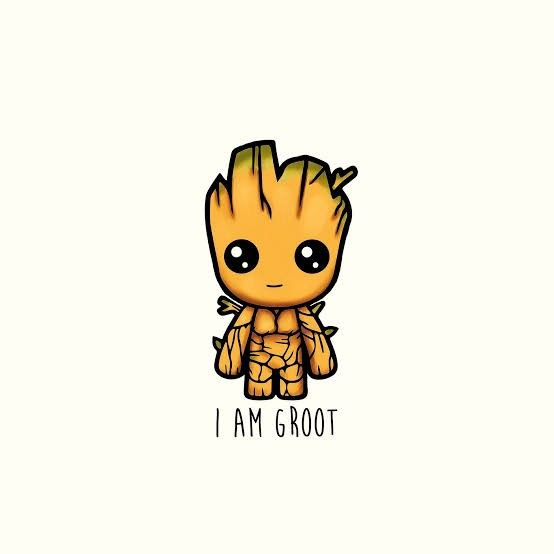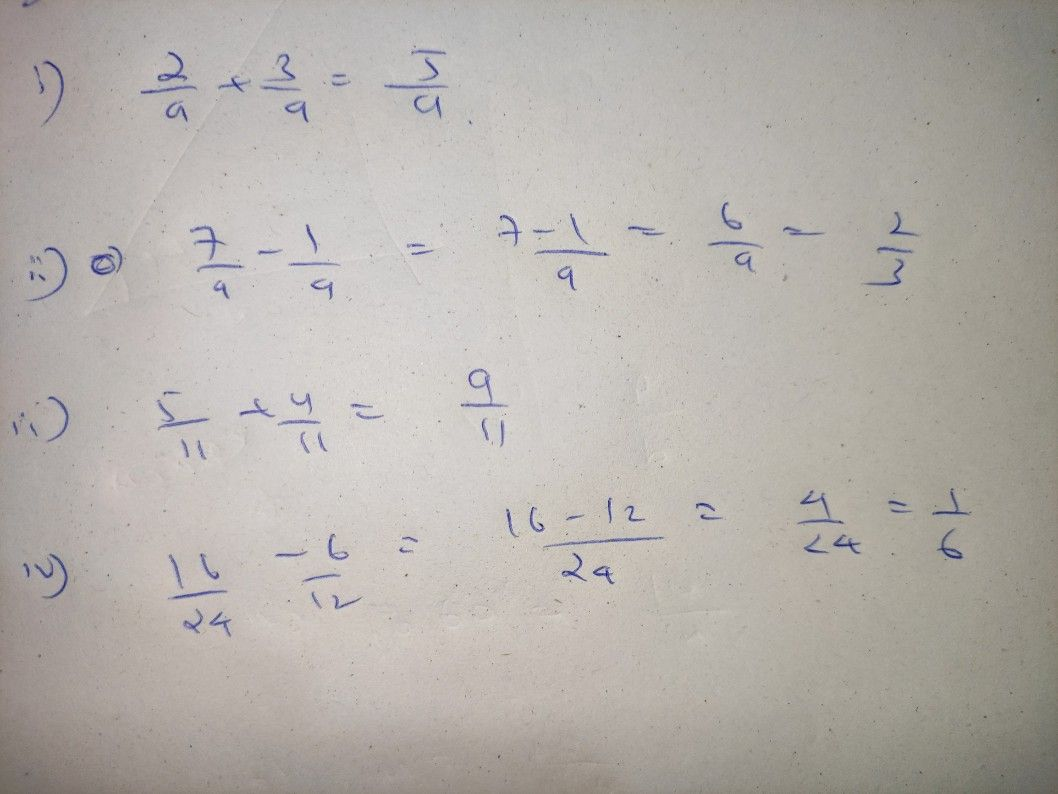Symbol
ProblemWhat's More $A1$ Perform the indicated operations and express your answer in simplest form. $1$ $\dfrac {2} {9}+\dfrac {3} {5}$ $2.\dfrac {7} {9}-\dfrac {1} {9}$ $3$ $\dfrac {5} {11}+\dfrac {4} {11}$ $4$ $\dfrac {16} {24}$ $\dfrac {6} {12}$ $5$ $\dfrac {7} {20}+\dfrac {3} {8}$ $6$ $\dfrac {4} {9}$ $1$
7th-9th grade
Calculus
Search count: 113
SolutionQanda teacher - Anwesha